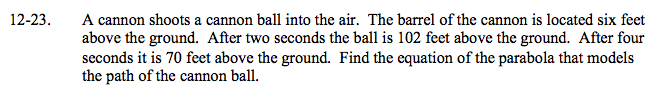### Home > A2C > Chapter 12 > Lesson 12.1.1 > Problem12-23

12-23.Identify the points that this information gives you.

(0, 6) (2, 102) (4, 70)

Use those points with the general equation of a parabola to create and solve a system of equations.

(6) = A(0)2 + B(0) + C
(102) = A(2)2 + B(2) + C
(70) = A(4)2 + B(4) + C

h(t) = −16t2 + 80t + 6